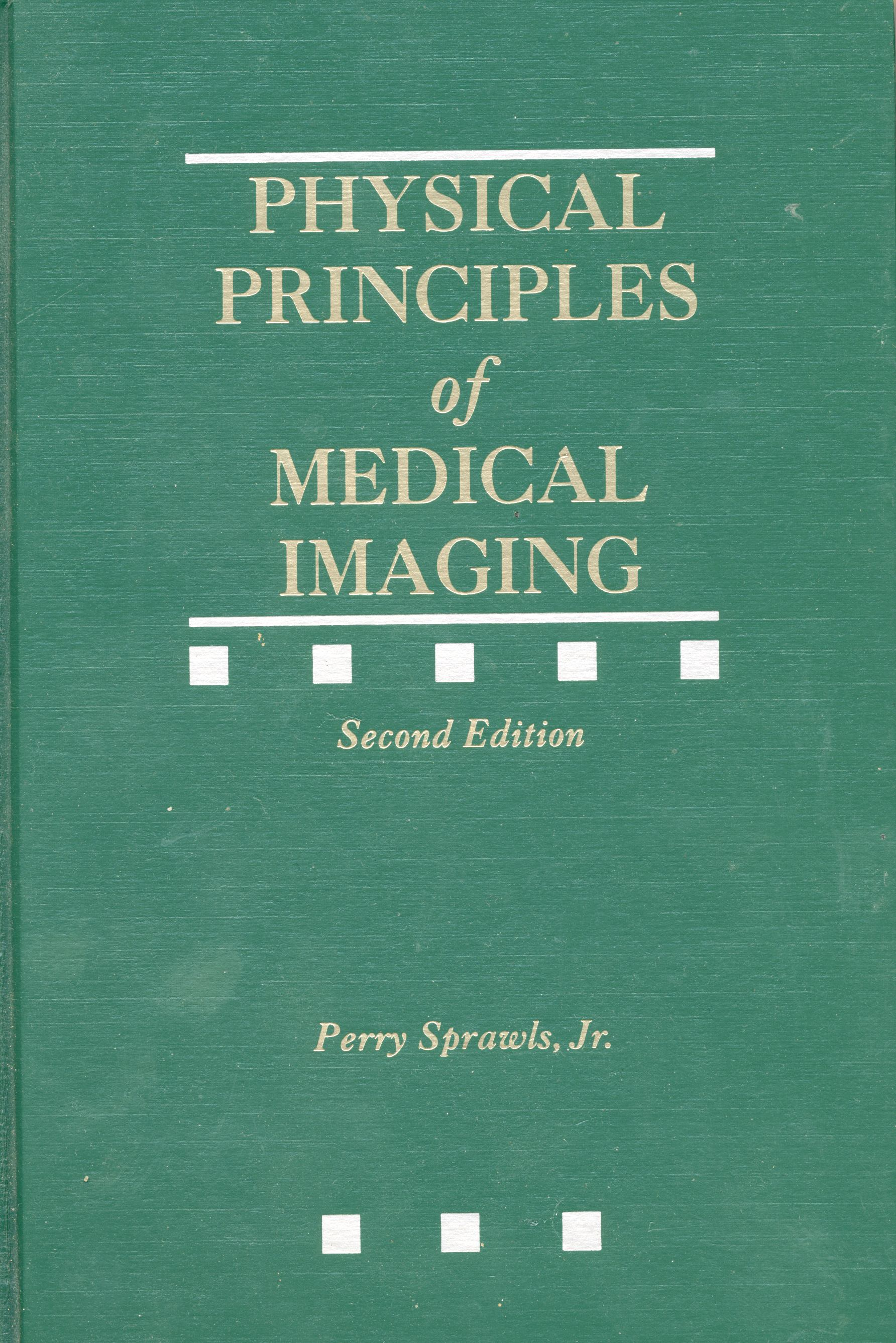Ultrasound Characteristics, Production, Interactions, and Control

 Outline Mind Map Learning Objectives Teaching Visuals Online Module Text Reference

 Learning Objectives Name and describe the basic physical characteristics of an ultrasound pulse that have an effect on it's imaging properties.  Describe the basic function of a transducer and how it forms  an ultrasound pulse.  State the range of ultrasound frequencies used for diagnostic procedures and give examples of clinical applications using the different frequencies.  Explain how the operator changes the ultrasound frequency in a typical imaging system.  Discuss the concept of ultrasound intensity, name the units, and state a typical value for a diagnostic imaging transducer.  Identify the physical factors which determine the velocity of ultrasound.  Discuss the physical factors that determine ultrasound wavelength and its significance in imaging.  Describe the general relationship between wavelength and image quality.  Describe the factors that determine the physical dimensions (length and width) of an ultrasound pulse.  Describe the physical conditions in the body that produce ultrasound reflections or echoes.  Describe the factors that determine the intensity of a reflected pulse.  Identify anatomical sites that tend to give strong reflections.  Briefly describe the physical process of ultrasound attenuation and loss of energy as a pulse moves through tissue.  Identify the three physical factors that determine the total attenuation of an ultrasound pulse passing through a section of tissue.  State the approximate ultrasound attenuation coefficient for:           a.  water                b.  soft tissue                   c.  air                d.  bone  Describe how ultrasound frequency relates to both image quality and tissue penetration.  Identify the factors that determine ultrasound velocity and state the approximate velocity value in tissue Explain the significance of velocity in ultrasound imaging and where it must be taken into consideration.  Identify the specific physical quantity and units that are used to express the rate at which ultrasound energy is imparted to a patient's body.  Explain the use of the unit decibel (dB) in ultrasound imaging.  State the ratio equivalence of 1, 3, and 6 dB's.  Calculate the approximate attenuation for 2.5 MHz ultrasound passing through 5 cm of soft tissue.   Describe the general effect of ultrasound attenuation on image formation.  Describe and name the feature and controls of an ultrasound system that is used to compensate for tissue attenuation.Text Reference Physical Principles of Medical Imaging, 2nd Ed Chapter 25 Medical Physics Publishing,  http://www.medicalphysics.org